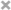# Xiuchuan Zhang

Personal Website

This is Xiuchuan's personal website.
I plan to post some of my current learning and review notes on it.
If you have any questions or suggestions, welcome to comment in my posts.

Most notes and code are from:
Embeddings

## Embedding layers

Using tf.keras API
Embeddings are a technique that enable deep neural nets to work with sparse categorical variables

### Set up

# Set up. Import libraries and load dataframes for Moivelens data
import numpy as np
import pandas as pd
from matplotlib import pyplot as plt
import tensorflow as tf
from tensorflow import keras
import os
import random

# Set random seeds for reproducibility
tf.set_random_seed(1); np.random.seed(1); random.seed(1)

input_dir = '../input'
ratings_path = os.path.join(input_dir, 'rating.csv')
ratings_df = pd.read_csv(ratings_path, usecols = ['userId','moiveId','rating','y'])

# Merge two dataframes
df = ratings_df.merge(movies_df, on = 'movieId').sort_values(by='userId')
df = df.sample(frac=1, random_state=1) #shuffle
df.sample(5, random_state=1)

n_movies = len(df.movieId.unique())
n_users = len(df.userId.unique())
print("{1:,} distinct users rated {0:,} different movies (total ratings = {2:,})".format(n_movies, n_users, len(df),))


This code will show that ‘138,493 distinct users rated 26,744 different movies (total ratings = 20,000,263)’, userId and movieId are both sparse categorical variables, they have many possible values.

### Rating prediction model in Keras

• Bad ideas: keras.Sequential
1. Use ids as numerical inputs
• the numerical values is meaningless
2. Use ids as categorical inputs
• One-hot encoded doing matrix multiplication so it makes inefficiency
• One-hot encoded only good on small number of possible values• Good idea: Embedding layers keras.Model
• Here is the code:
python
hidden_units = (32,4)
movie_embedding_size = 8
user_embedding_size = 8

# Each instance will consist of two inputs: a single user id, and a single movie id
user_id_input = keras.Input(shape=(1,), name = ‘user_id’)
movie_id_input = keras.Imput(shape=(1,), name = ‘movie_id’)
user_embedded = keras.layers.Embedding(df.userId.max()+1, user_embedding_size, input_length=1, name=’user_embedding’)(user_id_input)
movie_embedded = keras.layers.Embedding(df.movieId.max()+1, movie_embedding_size, input_length=1, name=’movie_embedding’)(movie_id_input)

# Concatenate the embeddings (and remove the useless extra dimension)
concatenated = keras.layers.Concatenate()([user_embedded, movie_embedded])
out = keras.layers.Flatten()(concatenated)

# Add one or more hidden layers
for n_hidden in hidden_units:
out = keras.layers.Dense(n_hidden, activation=’relu’)(out)

# A single output: our predicted rating
out = keras.layers.Dense(1, activation=’linear’, name=’prediction’)(out)

model = keras.Model( input = [useer_id_input, movie_id_input], outputs = out, )
model.summary(line_length = 88)


- Minimize squared error ('MSE') [tf.train]
python
model.compile(
# They seem to be much slower on problems like this, because they don't efficiently handle sparse gradient updates.
loss = 'MSE',
metrics = ['MAE'],
)


Support